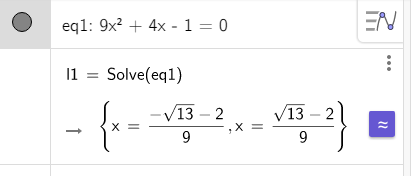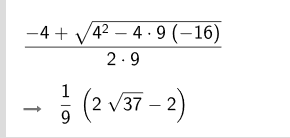Wrecked shared this question 2 years ago

I'm trying to teach GED students the quadratic formula remotely. For the test, they use a Texas Instruments calculator but they do not have the calculators at home. The TI calculator leaves the answers with the radical (See attached example). Your calculator solves the problem. Is there any setting so that I can use your calculator and leave the radical?1

If you type the equation in the inputfield, enter, click on the More button and choose the option solve, the solutions will appear in the algebra in radicals. You can toggle between radicals and decimals with the blue button.

This is as well in GG6, webversion and apps. In GG5 you can type the Sole command yourself manually1

Thank you. What if I need the students to input everything into the formula in the calculator and then solve? Like below:

Files: form.jpg1

Try the Substitute() command, or they can just type it1

I'm not sure I understand what you mean. Where would I go from here?1

Got it. Thanks!1

use CAS ? https://www.geogebra.org/cas1

I'll give that a try! I don't see a fraction button. How do I make a fraction?2

use /

(-4+sqrt(4²-4*9(-16))/(2*9)